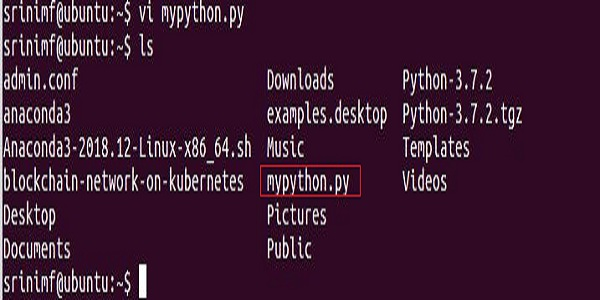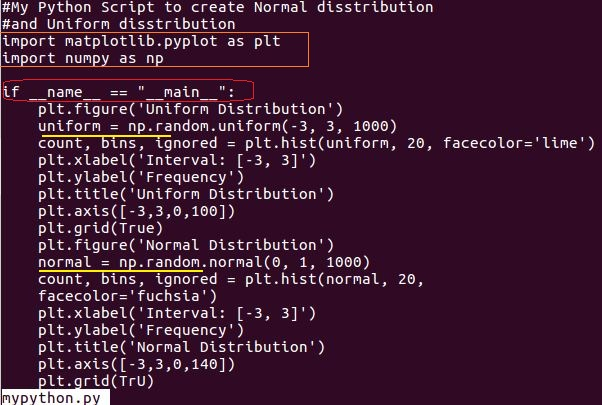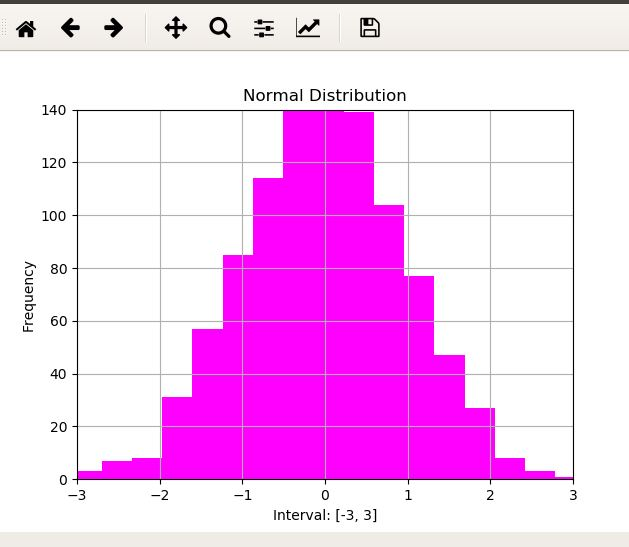### How to Check Column Nulls and Replace: PandasHere is a post that shows how to count Nulls and replace them with the value you want in the Pandas Dataframe. We have explained the process in two steps - Counting and Replacing the Null values. Count null values (column-wise) in Pandas ## count null values column-wise null_counts = df.isnull(). sum() print(null_counts) ``` Output: ``` Column1    1 Column2    1 Column3    5 dtype: int64 ``` In the above code, we first create a sample Pandas DataFrame `df` with some null values. Then, we use the `isnull()` function to create a DataFrame of the same shape as `df`, where each element is a boolean value indicating whether that element is null or not. Finally, we use the `sum()` function to count the number of null values in each column of the resulting DataFrame. The output shows the count of null values column-wise. to count null values column-wise: ``` df.isnull().sum() ``` ##Code snippet to count null values row-wise: ``` df.isnull().sum(axis=1) ``` In the above code, `df` is the Panda

# Data science: Simple Project to Practice

I want to share with you how to use Python for your Data science or analytics Projects. Many programmers struggle to learn Data science because they do not know where to start. You can get hands-on if you start with a mini-project.

I have used Ubuntu Operating System for this project. You Need dual skills; Learning and Apply knowledge to become a data scientist. In Data science you need to learn and apply your knowledge.After engineering, you can go for M Tech Degree.

You can become a real engineer if you apply engineering principles. So Data science also the same.

## Data Visualization in Python is my simple project

Importance of Data

Data is a precious resource in resolving Machine Learning and Data Science Problems.

Define first what is your problem.
1. Collect Data
2.  Wrangle the Data and Clean it.
3. Visualize the Patterns
In the olden days, you might be studied a subject called Statistical Analysis.

In this subject, you need to study the actual problem and collect the data in a notebook.

Let us say when there were no computers in the olden days, people use paper and notebooks to collect and analyze data.

After that, they use pencil and graph paper and draw the charts based on selected data.

It is time consuming and laborious process. Finally based on the data visualization people correct the process.

The same concept you can see in current data science projects.

Related Posts

### Make sure These Steps Completed

1. Install Ubuntu on a Virtual Machine
2.  Install Python 3.7X
3. Install Anaconda Python - Which contains all the packages that you need for Data science projects.
'ls' Command gives the Script I created for this Project### How to View Python Script using less command### 5 Key Points to Remember

1. Import command you can use to import packages.
2. matplotlib is a package. This you need to draw a plot.
3. Numpy is required to use all mathematical and scientific calculations. So I imported Numpy.
4. The 'as' command is an alias. So that you can save a lot of coding time.
5. I have drawn two plots. One is uniform and the other one in normal
Why I used if__name__=="__main__":

The real meaning is that the script is running under the main().

Also the module mypython.py, you can run as Standalone or you can call this module using import command from another script.

In Python, you need to create modules using the .py extension.

Some people say as scripts and other people say as Modules.

All Python documents and Standard textbooks using the word Python module. So you also can use it.

You May Also Like: Story of  Python name and main

### How to Execute mypython.py in Python Console

\$python mypython.py

## Two Plots I have Drawn: Normal and Uniform Distribution

1). Normal Distribution2). Uniform Distribution### Summary

Try today as this is a simple project. In realtime, a data analyst role is to deal with data and charts. I am sure you can begin your data analyst career with this project.

1.good information